# RT-Thread 互补滤波器 (STM32 + 6 轴 IMU)

07/11 20:10

Github - STM32 IMU 互补滤波器 (RT-Thread)：https://github.com/wuhanstudio/stm32-imu-filter

## IMU 传感器 (Inertial Measurement Unit)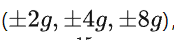，默认是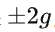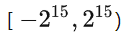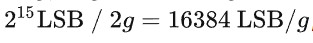double aSensitivity = 16384;accel_x = accel_x / aSensitivity;accel_y = accel_y / aSensitivity;accel_z = accel_z / aSensitivity;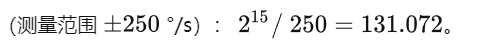double gSensitivity = 131;gyrX = gyro_x / gSensitivity;gyrY = gyro_y / gSensitivity;gyrZ = gyro_z / gSensitivity;

## 互补滤波器 (Complementary Filter)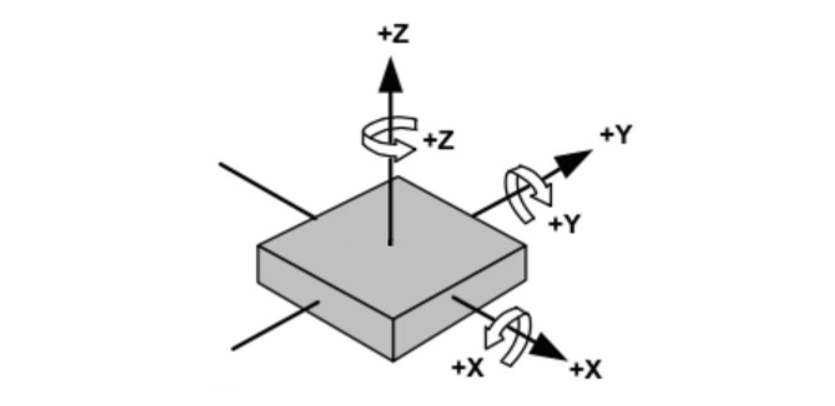### 陀螺仪估计姿态

// angles based on gyro (deg/s)gx = gx + gyrX * TIME_STEP_MS / 1000;gy = gy + gyrY * TIME_STEP_MS / 1000;gz = gz + gyrZ * TIME_STEP_MS / 1000;

### 加速度计估计姿态

// angles based on accelerometerax = atan2(accelY, accelZ) * 180 / M_PI;                                     // rollay = atan2(-accelX, sqrt( pow(accelY, 2) + pow(accelZ, 2))) * 180 / M_PI;    // pitch

### 互补滤波器

// complementary filtergx = gx * 0.96 + ax * 0.04;gy = gy * 0.96 + ay * 0.04;

## 总结

// angles based on gyro (deg/s)gx = gx + gyrX * TIME_STEP_MS / 1000;gy = gy + gyrY * TIME_STEP_MS / 1000;gz = gz + gyrZ * TIME_STEP_MS / 1000;// angles based on accelerometerax = atan2(accelY, accelZ) * 180 / M_PI;                                     // rollay = atan2(-accelX, sqrt( pow(accelY, 2) + pow(accelZ, 2))) * 180 / M_PI;    // pitch// complementary filtergx = gx * 0.96 + ax * 0.04;gy = gy * 0.96 + ay * 0.04;

## References

• https://github.com/mattzzw/Arduino-mpu6050👇👇👇 点击阅读原文查看近期赛事0 评论
0 收藏
0